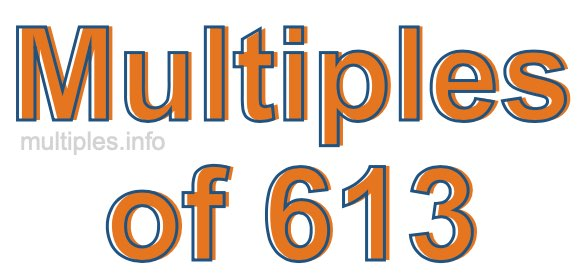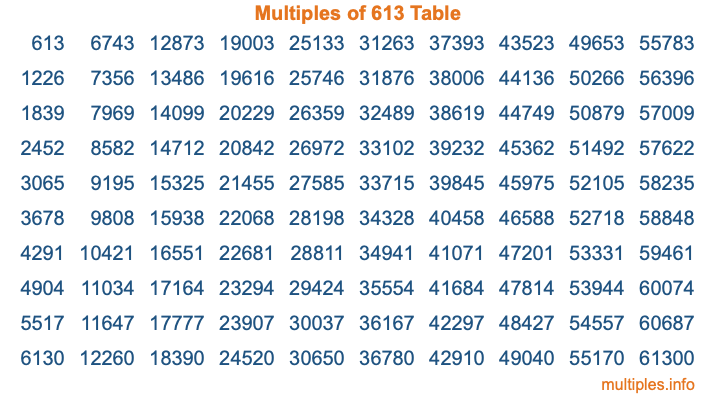Multiples of 613Welcome to the Multiples of 613 page. Here we will first teach you everything you will ever need to know about the multiples of 613, and then give you a study guide summary of everything we taught you to make sure you remember it all. Use this page to look up facts and learn information about the multiples of 613. This page will make you a multiples of six hundred thirteen expert!

Definition of Multiples of 613
Multiples of 613 are all the numbers that when divided by 613 equal an integer. Each of the multiples of 613 are called a multiple. A multiple of 613 is created by multiplying 613 by an integer.

Therefore, to create a list of multiples of 613, you start with 1 multiplied by 613, then 2 multiplied by 613, then 3 multiplied by 613, and so on for as long as you want. Thus, the list of the first five multiples of 613 is 613, 1226, 1839, 2452, and 3065. To see a larger list of multiples of 613, see the printable image of Multiples of 613 further down on this page. We also have a category where you can choose any nth multiple of 613.

Multiples of 613 Checker
The Multiples of 613 Checker below checks to see if any number of your choice is a multiple of 613. In other words, it checks to see if there is any number (integer) that when multiplied by 613 will equal your number. To do that, we divide your number by 613. If the the quotient is an integer, then your number is a multiple of 613.

Is  a multiple of 613?

Least Common Multiple of 613 and ...
A Least Common Multiple (LCM) is the lowest multiple that two or more numbers have in common. This is also called the smallest common multiple or lowest common multiple and is useful to know when you are adding our subtracting fractions. Enter one or more numbers below (613 is already entered) to find the LCM.

Check out our LCM Calculator if you need more details about the Least Common Multiple or if you need the LCM for different numbers for adding and subtraction fractions.

nth Multiple of 613
As we stated above, 613 is the first multiple of 613, 1226 is the second multiple of 613, 1839 is the third multiple of 613, and so on. Enter a number below to find the nth multiple of 613.

th multiple of 613

Multiples of 613 vs Factors of 613
613 is a multiple of 613 and a factor of 613, but that is where the similarities end. All postive multiples of 613 are 613 or greater than 613. All positive factors of 613 are 613 or less than 613.

Below is the beginning list of multiples of 613 and the factors of 613 so you can compare:

Multiples of 613: 613, 1226, 1839, 2452, 3065, etc.

Factors of 613: 1, 613

As you can see, the multiples of 613 are all the numbers that you can divide by 613 to get a whole number. The factors of 613, on the other hand, are all the whole numbers that you can multiply by another whole number to get 613.

It's also interesting to note that if a number (x) is a factor of 613, then 613 will also be a multiple of that number (x).

Multiples of 613 vs Divisors of 613
The divisors of 613 are all the integers that 613 can be divided by evenly. Below is a list of the divisors of 613.

Divisors of 613: 1, 613

The interesting thing to note here is that if you take any multiple of 613 and divide it by a divisor of 613, you will see that the quotient is an integer.

Multiples of 613 Table
Below is an image of the first 100 multiples of 613 in a table. The table is in chronological order, column by column. The first column has the first ten multiples of 613, the second column has the next ten multiples of 613, and so on.The Multiples of 613 Table is also referred to as the 613 Times Table or Times Table of 613. You are welcome to print out our table for your studies.

Negative Multiples of 613
Although not often discussed or needed in math, it is worth mentioning that you can make a list of negative multiples of 613 by multiplying 613 by -1, then by -2, then by -3, and so on, to get the following list of negative multiples of 613:

-613, -1226, -1839, -2452, -3065, etc.

Multiples of 613 Summary
Below is a summary of important Multiples of 613 facts that we have discussed on this page. To retain the knowledge on this page, we recommend that you read through the summary and explain to yourself or a study partner why they hold true.

There are an infinite number of multiples of 613.

A multiple of 613 divided by 613 will equal a whole number.

613 divided by a factor of 613 equals a divisor of 613.

The nth multiple of 613 is n times 613.

The largest factor of 613 is equal to the first positive multiple of 613.

613 is a multiple of every factor of 613.

613 is a multiple of 613.

A multiple of 613 divided by a divisor of 613 equals an integer.

613 divided by a divisor of 613 equals a factor of 613.

Any integer times 613 will equal a multiple of 613.

Multiples of a Number
Here you can get the multiples of another number, all with the same attention to detail as we did for multiples of 613 on this page.

Multiples of
Multiples of 614
Did you find our page about multiples of six hundred thirteen educational? Do you want more knowledge? Check out the multiples of the next number on our list!